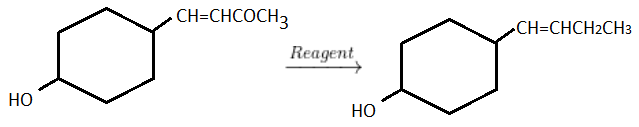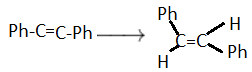# JEE Main Hydrocarbons Previous Year Questions with Solutions

Hydrocarbons Previous Year Questions with Solutions are given here. Hydrocarbons are organic compounds that are made up of only two kinds of atoms, carbon and hydrogen. These are colourless gases that have very weak odours. These can be generally classified into aliphatic and alicyclic hydrocarbons. The study of hydrocarbons can provide insight into the chemical properties of other functional groups and their preparation. Propane and butane are hydrocarbons that are used for commercial fuel purposes in the form of Liquefied Petroleum Gas (LPG). This article helps students to learn the previous year questions from hydrocarbons and score higher rank for the JEE exam.

The important topics related to hydrocarbons are classification, properties, preparation and uses. As far as the JEE exam is concerned, hydrocarbon is an important topic. This article gives an idea of what type of questions can be expected from this topic. BYJU’S provides solutions designed by our experts. Students can easily download the solutions in PDF format for free.

## JEE Main Chemistry Hydrocarbons Previous Year Questions With Solutions

1. The gas liberated by the electrolysis of Dipotassium succinate solution is :

(1) Ethyne

(2) Ethene

(3) Propene

(4) Ethane

Solution:

(CH2COO)2 → CH2 = CH2 + 2CO2 (g) + 2e

2H2O + 2e → 2OH + H2 (g)

So the gas generated during electrolysis of Dipotassium succinate solution is ethene.

Hence option (2) is the answer.

2. Which one of the following classes of compounds is obtained by polymerization of acetylene?

(1) Poly-ene

(2) Poly-yne

(3) Poly-amide

(4) Poly-ester

Solution:

nHC ≡ CH → ( CH =CH)n poly-yne

Hence option (2) is the answer.

3. One mole of a symmetrical alkene on ozonolysis gives two moles of an aldehyde having a molecular mass of 44 u. The alkene is:-

(1) Ethene

(2) Propene

(3) 1-Butene

(4) 2-Butene

Solution:

2-Butene on ozonolysis gives 2 moles of acetaldehyde with the molecular mass of 44gm/mol.

Hence option (4) is the answer.

4. Which branched chain isomer of the hydrocarbon with molecular mass 72 u gives only one isomer of mono substituted alkyl halide?

(1) Neopentane

(2) Isohexane

(3) Neohexane

(4) Tertiary butyl chloride

Solution:

Molecular mass indicates that it is pentane. Neopentane can only form one mono substituted alkyl halide as all the hydrogens are equivalent in neopentane.

Hence option (1) is the answer.

5. Ozonolysis of an organic compound gives formaldehyde as one of the products. This confirms the presence of:-

(1) an isopropyl group

(2) an acetylenic triple bond

(3) two ethylenic double bonds

(4) a vinyl group

Solution:

In Ozonolysis, the presence of the vinyl group gives formaldehyde as one of the products.

Hence option (3) is the answer.

6. In the given transformation, which of the following is the most appropriate reagent?(1) NaBH4

(2) NH2-NH2, OH

(3) Zn – Hg / HCl

(4) Na, Liq.NH3

Solution:

In NH2-NH2, OH, the medium is basic so it will not have any effect on the OH group in the reagent.

Hence option (2) is the answer.

7. The number and type of bonds in C22- ion in CaC2 are:

(1) Two σ bonds and one π – bond

(2) Two σ bonds and two π – bonds

(3) One σ bond and two π – bonds

(4) One σ bond and one π bond

Solution:

Ca+2 [C≡C] -2

One σ bond and two π – bonds are there in C22- ion in CaC2.

Hence option (3) is the answer.

8. In the hydroboration – oxidation reaction of propene with diborane, H2O2 and NaOH, the organic compound formed is :

(1) CH3CH2CH2OH

( 2) (CH3)3COH

(3) CH3CHOHCH3

(4) CH3CH2OH

Solution:

The hydroboration–oxidation reaction is a two-step hydration reaction that converts an alkene into alcohol. It is an anti-Markovnikov reaction. The organic compound formed in the hydroboration – oxidation reaction of propene with diborane, H2O2 and NaOH is CH3CH2CH2OH.

Hence option (1) is the answer.

9. Which one of the following has the minimum boiling point?

(1) n-Butane

(2) 1-Butyne

(3) 1-Butene

(4) Isobutene

Solution:

Among the isomeric alkanes, the normal isomer has a higher boiling point than the branched-chain isomer. The higher the branching of the chain, the lower is the boiling point. The n-alkanes have more surface area in comparison to branched-chain isomers. So, intermolecular forces are weaker in branched-chain isomers. Hence they have lower boiling points in comparison to straight-chain isomers.

Hence option (4) is the answer.

10. The gas evolved on heating CH3MgBr in methanol is

(1) methane

(2) ethane

(3) propane

(4) HBr

Solution:

The gas evolved on heating Methyl magnesium bromide in methanol is methane.

CH3-O-H + CH3MgBr → CH3-H + Ch3-OMgBr

Hence option (1) is the answer.

11. The reagent needed for convertingis

(1) H2 / lindlar Cat.

(2) Catalytic Hydrogenation

(3) LiAlH4

(4) Li/ NH3

Solution:

Li/NH3 is the birch reagent. It reduces the alkyne to trans alkene.

Hence option (4) is the answer.

12. In the presence of peroxide, HCl and HI do not give anti-Markovnikov’s addition to alkenes because

(1) All the steps are exothermic in HCl and HI

(2) One of the steps is endothermic in HCl and HI

(3) HCl is oxidizing and the HI is reducing

(4) Both HCl and HI are strong acids

Solution:

Bromides will take part in radical based reactions in the presence of organic peroxides. Fission of the peroxide O-O linkage causes a Br radical which behaves differently to Bromide, by adding to the less substituted side of the alkene(anti – markovnikov). HI and HCl does not do this for energetic reasons. The addition of Cl and I radicals to the alkene in an anti-markovnikov fashion is an endothermic reaction. Hence it is unfavourable. All hydrogen halides will add according to the markovnikov rule, without the presence of peroxide,

Hence option (2) is the answer.

13. Polysubstitution is a major drawback in

(a) Reimer-Tiemann reaction

(b) Friedel-Crafts acylation

(c) Friedel-Crafts alkylation

(d) Acetylation of aniline.

Solution:

The product obtained is more activated in Friedel-Crafts alkylation and thus, polysubstitution will take place.

Hence option (3) is the answer.

14. Which one of the following is reduced with zinc and hydrochloric acid to give the corresponding hydrocarbon?

(1) Ethyl acetate

(2) Acetic acid

(3) Acetamide

(4) Butan-2-one

Solution:

Butan-2-one will get reduced into butane when treated with zinc and hydrochloric acid following Clemmensen reaction whereas Zn/HCl do not reduce ester, acid and amide.

Hence option (4) is the answer.

15. The hydrocarbon which can react with sodium in liquid ammonia is

(1) CH3CH2C ≡ CCH2CH3

(2) CH3CH2CH2C ≡ CCH2CH2CH3

(3) CH3CH2C ≡ CH

(4) CH3CH ≡ CHCH3

Solution:

Terminal alkynes have acidic hydrogen. Terminal alkynes react with sodium in liquid ammonia to give ionic compounds.

Hence option (3) is the answer.